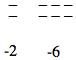### Home > MC1 > Chapter 2 > Lesson 2.3.1 > Problem2-107

2-107.

Show the numbers of + and − pieces represented by each expression below. Sketch each model and state the number that it represents.

1. $(−2)+(−6)$

Sketch a model of the integers.From the model, determine what the expression represents.

For more help, look at the Math Notes in Lesson 2.3.1.

$-8$

1. $(−2)+4+(−2)$

Use part (a) as a guide for this problem. Remember to look for and eliminate zeros.

Draw a diagram to model this expression. This expression represents 0.

1. $5+(−8)$

Use part (a) as a guide for solving this problem.